Documentation

### This is machine translation

Mouseover text to see original. Click the button below to return to the English version of the page.

## Nonlinear State Estimation Using Unscented Kalman Filter and Particle Filter

This example shows how to use the unscented Kalman filter and particle filter algorithms for nonlinear state estimation for the van der Pol oscillator.

This example also uses the Signal Processing Toolbox™.

### Introduction

Control System Toolbox™ offers three commands for nonlinear state estimation:

• `extendedKalmanFilter`: First-order, discrete-time extended Kalman filter

• `unscentedKalmanFilter`: Discrete-time unscented Kalman filter

• `particleFilter`: Discrete-time particle filter

A typical workflow for using these commands is as follows:

1. Model your plant and sensor behavior.

2. Construct and configure the `extendedKalmanFilter`, `unscentedKalmanFilter` or `particleFilter` object.

3. Perform state estimation by using the `predict` and `correct` commands with the object.

4. Analyze results to gain confidence in filter performance

5. Deploy the filter on your hardware. You can generate code for these filters using MATLAB Coder™.

This example first uses the `unscentedKalmanFilter` command to demonstrate this workflow. Then it demonstrates the use of particleFilter.

### Plant Modeling and Discretization

The unscented Kalman filter (UKF) algorithm requires a function that describes the evolution of states from one time step to the next. This is typically called the state transition function. unscentedKalmanFilter supports the following two function forms:

1. Additive process noise: $x\left[k\right]=f\left(x\left[k-1\right],u\left[k-1\right]\right)+w\left[k-1\right]$

2. Non-additive process noise: $x\left[k\right]=f\left(x\left[k-1\right],w\left[k-1\right],u\left[k-1\right]\right)$

Here f(..) is the state transition function, x is the state, w is the process noise. u is optional and represents additional inputs to f, for instance system inputs or parameters. u can be specified as zero or more function arguments. Additive noise means that the state and process noise is related linearly. If the relationship is nonlinear, use the second form. When you create the unscentedKalmanFilter object, you specify f(..) and also whether the process noise is additive or non-additive.

The system in this example is the van der Pol oscillator with mu=1. This 2-state system is described with the following set of nonlinear ordinary differential equations (ODE):

`$\begin{array}{l}{\underset{}{\overset{˙}{x}}}_{1}={x}_{2}\\ {\underset{}{\overset{˙}{x}}}_{2}=\left(1-{x}_{1}^{2}\right){x}_{2}-{x}_{1}\end{array}$`

Denote this equation as $\underset{}{\overset{˙}{x}}={f}_{c}\left(x\right)$, where $x=\left[{x}_{1};{x}_{2}\right]$. The process noise w does not appear in the system model. You can assume it is additive for simplicity.

unscentedKalmanFilter requires a discrete-time state transition function, but the plant model is continuous-time. You can use a discrete-time approximation to the continuous-time model. Euler discretization is one common approximation method. Assume that your sample time is ${T}_{s}$, and denote the continuous-time dynamics you have as $\underset{}{\overset{˙}{x}}={f}_{c}\left(x\right)$. Euler discretization approximates the derivative operator as $\underset{}{\overset{˙}{x}}\approx \frac{x\left[k+1\right]-x\left[k\right]}{{T}_{s}}$. The resulting discrete-time state-transition function is:

`$\begin{array}{ll}x\left[k+1\right]& =x\left[k\right]+{f}_{c}\left(x\left[k\right]\right)\phantom{\rule{0.2777777777777778em}{0ex}}{T}_{s}\\ & =f\left(x\left[k\right]\right)\end{array}$`

The accuracy of this approximation depends on the sample time ${T}_{s}$. Smaller ${T}_{s}$ values provide better approximations. Alternatively, you can use a different discretization method. For instance, higher order Runge-Kutta family of methods provide a higher accuracy at the expense of more computational cost, given a fixed sample time ${T}_{s}$.

Create this state-transition function and save it in a file named vdpStateFcn.m. Use the sample time ${T}_{s}=0.05s$. You provide this function to the `unscentedKalmanFilter` during object construction.

```addpath(fullfile(matlabroot,'examples','control','main')) % add example data type vdpStateFcn```
```function x = vdpStateFcn(x) % vdpStateFcn Discrete-time approximation to van der Pol ODEs for mu = 1. % Sample time is 0.05s. % % Example state transition function for discrete-time nonlinear state % estimators. % % xk1 = vdpStateFcn(xk) % % Inputs: % xk - States x[k] % % Outputs: % xk1 - Propagated states x[k+1] % % See also extendedKalmanFilter, unscentedKalmanFilter % Copyright 2016 The MathWorks, Inc. %#codegen % The tag %#codegen must be included if you wish to generate code with % MATLAB Coder. % Euler integration of continuous-time dynamics x'=f(x) with sample time dt dt = 0.05; % [s] Sample time x = x + vdpStateFcnContinuous(x)*dt; end function dxdt = vdpStateFcnContinuous(x) %vdpStateFcnContinuous Evaluate the van der Pol ODEs for mu = 1 dxdt = [x(2); (1-x(1)^2)*x(2)-x(1)]; end ```

### Sensor Modeling

unscentedKalmanFilter also needs a function that describes how the model states are related to sensor measurements. unscentedKalmanFilter supports the following two function forms:

1. Additive measurement noise: $y\left[k\right]=h\left(x\left[k\right],u\left[k\right]\right)+v\left[k\right]$

2. Non-additive measurement noise: $y\left[k\right]=h\left(x\left[k\right],v\left[k\right],u\left[k\right]\right)$

h(..) is the measurement function, v is the measurement noise. u is optional and represents additional inputs to h, for instance system inputs or parameters. u can be specified as zero or more function arguments. You can add additional system inputs following the u term. These inputs can be different than the inputs in the state transition function.

For this example, assume you have measurements of the first state ${x}_{1}$ within some percentage error:

`$y\left[k\right]={x}_{1}\left[k\right]\phantom{\rule{0.2777777777777778em}{0ex}}\left(1+v\left[k\right]\right)$`

This falls into the category of non-additive measurement noise because the measurement noise is not simply added to a function of states. You want to estimate both ${x}_{1}$ and ${x}_{2}$ from the noisy measurements.

Create this state transition function and save it in a file named vdpMeasurementNonAdditiveNoiseFcn.m. You provide this function to the `unscentedKalmanFilter` during object construction.

`type vdpMeasurementNonAdditiveNoiseFcn`
```function yk = vdpMeasurementNonAdditiveNoiseFcn(xk,vk) % vdpMeasurementNonAdditiveNoiseFcn Example measurement function for discrete % time nonlinear state estimators with non-additive measurement noise. % % yk = vdpNonAdditiveMeasurementFcn(xk,vk) % % Inputs: % xk - x[k], states at time k % vk - v[k], measurement noise at time k % % Outputs: % yk - y[k], measurements at time k % % The measurement is the first state with multiplicative noise % % See also extendedKalmanFilter, unscentedKalmanFilter % Copyright 2016 The MathWorks, Inc. %#codegen % The tag %#codegen must be included if you wish to generate code with % MATLAB Coder. yk = xk(1)*(1+vk); end ```

### Unscented Kalman Filter Construction

Construct the filter by providing function handles to the state transition and measurement functions, followed by your initial state guess. The state transition model has additive noise. This is the default setting in the filter, hence you do not need to specify it. The measurement model has non-additive noise, which you must specify through setting the `HasAdditiveMeasurementNoise` property of the object as `false`. This must be done during object construction. If your application has non-additive process noise in the state transition function, specify the `HasAdditiveProcessNoise` property as `false`.

```% Your initial state guess at time k, utilizing measurements up to time k-1: xhat[k|k-1] initialStateGuess = [2;0]; % xhat[k|k-1] % Construct the filter ukf = unscentedKalmanFilter(... @vdpStateFcn,... % State transition function @vdpMeasurementNonAdditiveNoiseFcn,... % Measurement function initialStateGuess,... 'HasAdditiveMeasurementNoise',false);```

Provide your knowledge of the measurement noise covariance

```R = 0.2; % Variance of the measurement noise v[k] ukf.MeasurementNoise = R;```

ProcessNoise property stores the process noise covariance. It is set to account for model inaccuracies and the effect of unknown disturbances on the plant. We have the true model in this example, but discretization introduces errors. This example did not include any disturbances for simplicity. Set it to a diagonal matrix with less noise on the first state, and more on the second state to reflect the knowledge that the second state is more impacted by modeling errors.

`ukf.ProcessNoise = diag([0.02 0.1]);`

### Estimation Using predict and correct Commands

In your application, the measurement data arriving from your hardware in real-time are processed by the filters as they arrive. This operation is demonstrated in this example by generating a set of measurement data first, and then feeding it to the filter one step at a time.

Simulate the van der Pol oscillator for 5 seconds with the filter sample time 0.05 [s] to generate the true states of the system.

```T = 0.05; % [s] Filter sample time timeVector = 0:T:5; [~,xTrue]=ode45(@vdp1,timeVector,[2;0]);```

Generate the measurements assuming that a sensor measures the first state, with a standard deviation of 45% error in each measurement.

```rng(1); % Fix the random number generator for reproducible results yTrue = xTrue(:,1); yMeas = yTrue .* (1+sqrt(R)*randn(size(yTrue))); % sqrt(R): Standard deviation of noise```

Pre-allocate space for data that you will analyze later

```Nsteps = numel(yMeas); % Number of time steps xCorrectedUKF = zeros(Nsteps,2); % Corrected state estimates PCorrected = zeros(Nsteps,2,2); % Corrected state estimation error covariances e = zeros(Nsteps,1); % Residuals (or innovations)```

Perform online estimation of the states x using the `correct` and `predict` commands. Provide generated data to the filter one time step at a time.

```for k=1:Nsteps % Let k denote the current time. % % Residuals (or innovations): Measured output - Predicted output e(k) = yMeas(k) - vdpMeasurementFcn(ukf.State); % ukf.State is x[k|k-1] at this point % Incorporate the measurements at time k into the state estimates by % using the "correct" command. This updates the State and StateCovariance % properties of the filter to contain x[k|k] and P[k|k]. These values % are also produced as the output of the "correct" command. [xCorrectedUKF(k,:), PCorrected(k,:,:)] = correct(ukf,yMeas(k)); % Predict the states at next time step, k+1. This updates the State and % StateCovariance properties of the filter to contain x[k+1|k] and % P[k+1|k]. These will be utilized by the filter at the next time step. predict(ukf); end```

### Unscented Kalman Filter Results and Validation

Plot the true and estimated states over time. Also plot the measured value of the first state.

```figure(); subplot(2,1,1); plot(timeVector,xTrue(:,1),timeVector,xCorrectedUKF(:,1),timeVector,yMeas(:)); legend('True','UKF estimate','Measured') ylim([-2.6 2.6]); ylabel('x_1'); subplot(2,1,2); plot(timeVector,xTrue(:,2),timeVector,xCorrectedUKF(:,2)); ylim([-3 1.5]); xlabel('Time [s]'); ylabel('x_2');```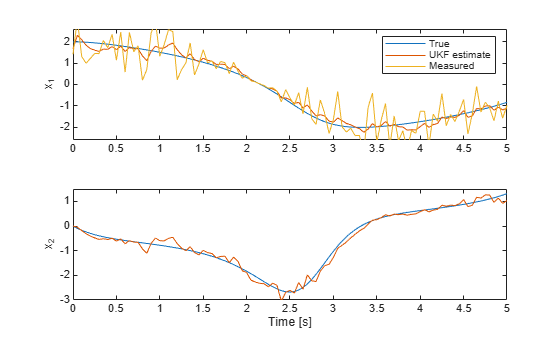The top plot shows the true, estimated, and the measured value of the first state. The filter utilizes the system model and noise covariance information to produce an improved estimate over the measurements. The bottom plot shows the second state. The filter is is successful in producing a good estimate.

The validation of unscented and extended Kalman filter performance is typically done using extensive Monte Carlo simulations. These simulations should test variations of process and measurement noise realizations, plant operating under various conditions, initial state and state covariance guesses. The key signal of interest used for validating the state estimation is the residuals (or innovations). In this example, you perform residual analysis for a single simulation. Plot the residuals.

```figure(); plot(timeVector, e); xlabel('Time [s]'); ylabel('Residual (or innovation)');```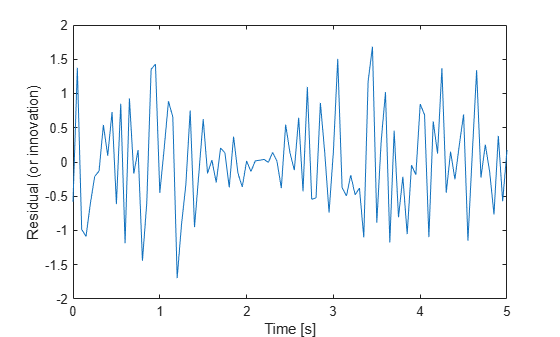The residuals should have:

1. Small magnitude

2. Zero mean

3. No autocorrelation, except at zero lag

The mean value of the residuals is:

`mean(e)`
```ans = -0.0012 ```

This is small relative to the magnitude of the residuals. The autocorrelation of the residuals can be calculated with the xcorr function in the Signal Processing Toolbox.

```[xe,xeLags] = xcorr(e,'coeff'); % 'coeff': normalize by the value at zero lag % Only plot non-negative lags idx = xeLags>=0; figure(); plot(xeLags(idx),xe(idx)); xlabel('Lags'); ylabel('Normalized correlation'); title('Autocorrelation of residuals (innovation)');```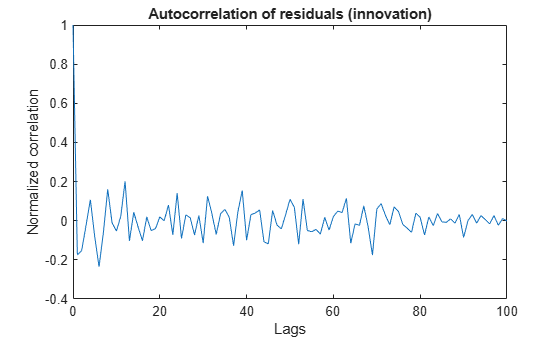The correlation is small for all lags except 0. The mean correlation is close to zero, and the correlation does not show any significant non-random variations. These characteristics increase confidence in filter performance.

In reality the true states are never available. However, when performing simulations, you have access to real states and can look at the errors between estimated and true states. These errors must satisfy similar criteria to the residual:

1. Small magnitude

2. Variance within filter error covariance estimate

3. Zero mean

4. Uncorrelated.

First, look at the error and the $1\sigma$ uncertainty bounds from the filter error covariance estimate.

```eStates = xTrue-xCorrectedUKF; figure(); subplot(2,1,1); plot(timeVector,eStates(:,1),... % Error for the first state timeVector, sqrt(PCorrected(:,1,1)),'r', ... % 1-sigma upper-bound timeVector, -sqrt(PCorrected(:,1,1)),'r'); % 1-sigma lower-bound xlabel('Time [s]'); ylabel('Error for state 1'); title('State estimation errors'); subplot(2,1,2); plot(timeVector,eStates(:,2),... % Error for the second state timeVector,sqrt(PCorrected(:,2,2)),'r', ... % 1-sigma upper-bound timeVector,-sqrt(PCorrected(:,2,2)),'r'); % 1-sigma lower-bound xlabel('Time [s]'); ylabel('Error for state 2'); legend('State estimate','1-sigma uncertainty bound',... 'Location','Best');```The error bound for state 1 approaches 0 at t=2.15 seconds because of the sensor model (MeasurementFcn). It has the form ${x}_{1}\left[k\right]*\left(1+v\left[k\right]\right)$. At t=2.15 seconds the true and estimated states are near zero, which implies the measurement error in absolute terms is also near zero. This is reflected in the state estimation error covariance of the filter.

Calculate what percentage of the points are beyond the 1-sigma uncertainty bound.

```distanceFromBound1 = abs(eStates(:,1))-sqrt(PCorrected(:,1,1)); percentageExceeded1 = nnz(distanceFromBound1>0) / numel(eStates(:,1)); distanceFromBound2 = abs(eStates(:,2))-sqrt(PCorrected(:,2,2)); percentageExceeded2 = nnz(distanceFromBound2>0) / numel(eStates(:,2)); [percentageExceeded1 percentageExceeded2]```
```ans = 1×2 0.1386 0 ```

The first state estimation errors exceed the $1\sigma$ uncertainty bound approximately 14% of the time steps. Less than 30% of the errors exceeding the 1-sigma uncertainty bound implies good estimation. This criterion is satisfied for both states. The 0% percentage for the second state means that the filter is conservative: most likely the combined process and measurement noises are too high. Likely a better performance can be obtained by tuning these parameters.

Calculate the mean autocorrelation of state estimation errors. Also plot the autocorrelation.

`mean(eStates)`
```ans = 1×2 -0.0103 0.0200 ```
```[xeStates1,xeStatesLags1] = xcorr(eStates(:,1),'coeff'); % 'coeff': normalize by the value at zero lag [xeStates2,xeStatesLags2] = xcorr(eStates(:,2),'coeff'); % 'coeff' % Only plot non-negative lags idx = xeStatesLags1>=0; figure(); subplot(2,1,1); plot(xeStatesLags1(idx),xeStates1(idx)); xlabel('Lags'); ylabel('For state 1'); title('Normalized autocorrelation of state estimation error'); subplot(2,1,2); plot(xeStatesLags2(idx),xeStates2(idx)); xlabel('Lags'); ylabel('For state 2');```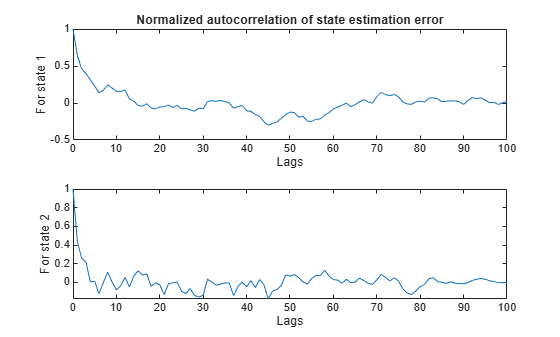The mean value of the errors is small relative to the value of the states. The autocorrelation of state estimation errors shows little non-random variations for small lag values, but these are much smaller than the normalized peak value 1. Combined with the fact that the estimated states are accurate, this behavior of the residuals can be considered as satisfactory results.

### Particle Filter Construction

Unscented and extended Kalman filters aim to track the mean and covariance of the posterior distribution of the state estimates by different approximation methods. These methods may not be sufficient if the nonlinearities in the system are severe. In addition, for some applications, just tracking the mean and covariance of the posterior distribution of the state estimates may not be sufficient. Particle filter can address these problems by tracking the evolution of many state hypotheses (particles) over time, at the expense of higher computational cost. The computational cost and estimation accuracy increases with the number of particles.

The particleFilter command in Control System Toolbox implements a discrete-time particle filter algorithm. This section walks you through constructing a particleFilter for the same van der Pol oscillator used earlier in this example, and highlights the similarities and differences with the unscented Kalman filter.

The state transition function you provide to particleFilter must perform two tasks. One, sampling the process noise from any distribution appropriate for your system. Two, calculating the time propagation of all particles (state hypotheses) to the next step, including the effect of process noise you calculated in step one.

`type vdpParticleFilterStateFcn`
```function particles = vdpParticleFilterStateFcn(particles) % vdpParticleFilterStateFcn Example state transition function for particle % filter % % Discrete-time approximation to van der Pol ODEs for mu = 1. % Sample time is 0.05s. % % predictedParticles = vdpParticleFilterStateFcn(particles) % % Inputs: % particles - Particles at current time. Matrix with dimensions % [NumberOfStates NumberOfParticles] matrix % % Outputs: % predictedParticles - Predicted particles for the next time step % % See also particleFilter % Copyright 2017 The MathWorks, Inc. %#codegen % The tag %#codegen must be included if you wish to generate code with % MATLAB Coder. [numberOfStates, numberOfParticles] = size(particles); % Time-propagate each particle % % Euler integration of continuous-time dynamics x'=f(x) with sample time dt dt = 0.05; % [s] Sample time for kk=1:numberOfParticles particles(:,kk) = particles(:,kk) + vdpStateFcnContinuous(particles(:,kk))*dt; end % Add Gaussian noise with variance 0.025 on each state variable processNoise = 0.025*eye(numberOfStates); particles = particles + processNoise * randn(size(particles)); end function dxdt = vdpStateFcnContinuous(x) %vdpStateFcnContinuous Evaluate the van der Pol ODEs for mu = 1 dxdt = [x(2); (1-x(1)^2)*x(2)-x(1)]; end ```

There are differences between the state transition function you supply to unscentedKalmanFilter and particleFilter. The state transition function you used for unscented Kalman filter just described propagation of one state hypothesis to the next time step, instead of a set of hypotheses. In addition, the process noise distribution was defined in ProcessNoise property of the unscentedKalmanFilter, just by its covariance. Particle filter can consider arbitrary distributions that may require more statistical properties to be defined. This arbitrary distribution and its parameters are fully defined in the state transition function you provide to the particleFilter.

The measurement likelihood function you provide to particleFilter must also perform two tasks. One, calculating measurement hypotheses from particles. Two, calculating the likelihood of each particle from the sensor measurement and the hypotheses calculated in step one.

`type vdpExamplePFMeasurementLikelihoodFcn`
```function likelihood = vdpExamplePFMeasurementLikelihoodFcn(particles,measurement) % vdpExamplePFMeasurementLikelihoodFcn Example measurement likelihood function % % The measurement is the first state. % % likelihood = vdpParticleFilterMeasurementLikelihoodFcn(particles, measurement) % % Inputs: % particles - NumberOfStates-by-NumberOfParticles matrix that holds % the particles % % Outputs: % likelihood - A vector with NumberOfParticles elements whose n-th % element is the likelihood of the n-th particle % % See also extendedKalmanFilter, unscentedKalmanFilter % Copyright 2017 The MathWorks, Inc. %#codegen % The tag %#codegen must be included if you wish to generate code with % MATLAB Coder. % Validate the sensor measurement numberOfMeasurements = 1; % Expected number of measurements validateattributes(measurement, {'double'}, {'vector', 'numel', numberOfMeasurements}, ... 'vdpExamplePFMeasurementLikelihoodFcn', 'measurement'); % The measurement is first state. Get all measurement hypotheses from particles predictedMeasurement = particles(1,:); % Assume the ratio of the error between predicted and actual measurements % follow a Gaussian distribution with zero mean, variance 0.2 mu = 0; % mean sigma = 0.2 * eye(numberOfMeasurements); % variance % Use multivariate Gaussian probability density function, calculate % likelihood of each particle numParticles = size(particles,2); likelihood = zeros(numParticles,1); C = det(2*pi*sigma) ^ (-0.5); for kk=1:numParticles errorRatio = (predictedMeasurement(kk)-measurement)/predictedMeasurement(kk); v = errorRatio-mu; likelihood(kk) = C * exp(-0.5 * (v' / sigma * v) ); end end ```

Now construct the filter, and initialize it with 1000 particles around the mean [2; 0] with 0.01 covariance. The covariance is small because you have high confidence in your guess [2; 0].

```pf = particleFilter(@vdpParticleFilterStateFcn,@vdpExamplePFMeasurementLikelihoodFcn); initialize(pf, 1000, [2;0], 0.01*eye(2));```

Optionally, pick the state estimation method. This is set by the StateEstimationMethod property of particleFilter, which can take the value 'mean' (default) or 'maxweight'. When StateEstimationMethod is 'mean', the object extracts a weighted mean of the particles from the Particles and Weights properties as the state estimate. 'maxweight' corresponds to choosing the particle (state hypothesis) as the state estimate. Alternatively, you can access Particles and Weights properties of the object and extract your state estimate via an arbitrary method of your choice.

`pf.StateEstimationMethod`
```ans = 'mean' ```

particleFilter lets you specify various resampling options via its ResamplingPolicy and ResamplingMethod properties. This example uses the default settings in the filter. See the particleFilter documentation for further details on resampling.

`pf.ResamplingMethod`
```ans = 'multinomial' ```
`pf.ResamplingPolicy`
```ans = particleResamplingPolicy with properties: TriggerMethod: 'ratio' SamplingInterval: 1 MinEffectiveParticleRatio: 0.5000 ```

Start the estimation loop. This represents measurements arriving over time, step by step.

```% Estimate xCorrectedPF = zeros(size(xTrue)); for k=1:size(xTrue,1) % Use measurement y[k] to correct the particles for time k xCorrectedPF(k,:) = correct(pf,yMeas(k)); % Filter updates and stores Particles[k|k], Weights[k|k] % The result is x[k|k]: Estimate of states at time k, utilizing % measurements up to time k. This estimate is the mean of all particles % because StateEstimationMethod was 'mean'. % % Now, predict particles at next time step. These are utilized in the % next correct command predict(pf); % Filter updates and stores Particles[k+1|k] end ```

Plot the state estimates from particle filter:

```figure(); subplot(2,1,1); plot(timeVector,xTrue(:,1),timeVector,xCorrectedPF(:,1),timeVector,yMeas(:)); legend('True','Particlte filter estimate','Measured') ylim([-2.6 2.6]); ylabel('x_1'); subplot(2,1,2); plot(timeVector,xTrue(:,2),timeVector,xCorrectedPF(:,2)); ylim([-3 1.5]); xlabel('Time [s]'); ylabel('x_2');```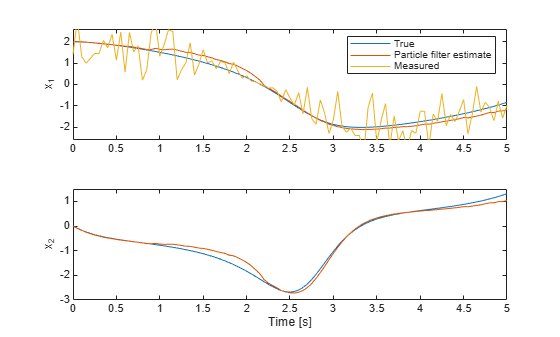The top plot shows the true value, particle filter estimate, and the measured value of the first state. The filter utilizes the system model and noise information to produce an improved estimate over the measurements. The bottom plot shows the second state. The filter is successful in producing a good estimate.

The validation of the particle filter performance involves performing statistical tests on residuals, similar to those that were performed earlier in this example for unscented Kalman filter results.

### Summary

This example has shown the steps of constructing and using an unscented Kalman filter and a particle filter for state estimation of a nonlinear system. You estimated states of a van der Pol oscillator from noisy measurements, and validated the estimation performance.

`rmpath(fullfile(matlabroot,'examples','control','main')) % remove example data`